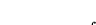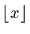Next: The Minimum Function Up: Mathematical Functions Previous: The Maximum Function

### Maximize Function

Calling Sequence:
MaximizeFunc(
f, r, tol)
MaximizeFunc(
f, r)
Parameters:
 f : procedure r :tol :Returns: A list of length 2 of the form [x, f(x)].

Synopsis: This function finds the maximum [x, f(x)] of a convex function f over a range r within an absolute accuracy of tol. If tol is not given as a parameter, the result is of machine accuracy.

Examples:

```> MaximizeFunc(x -> sin(x), 0..1);
> z := proc(x) return(x^2 - 3*x); end;
> MaximizeFunc(z, -2..0);
```